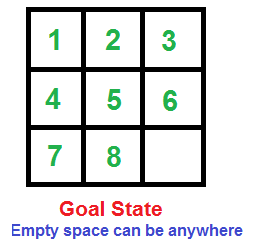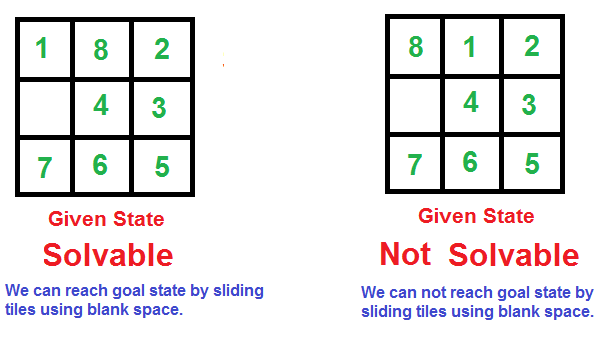Open in App
Not now

# How to check if an instance of 8 puzzle is solvable?

• Difficulty Level : Hard
• Last Updated : 26 Jul, 2022

### What is 8 puzzle?

Given a 3×3 board with 8 tiles (every tile has one number from 1 to 8) and one empty space. The objective is to place the numbers on tiles in order using the empty space. We can slide four adjacent (left, right, above and below) tiles into the empty space### How to find if given state is solvable?

Following are two examples, the first example can reach goal state by a series of slides. The second example cannot.Following is simple rule to check if a 8 puzzle is solvable.

It is not possible to solve an instance of 8 puzzle if number of inversions is odd in the input state.

In the examples given in above figure, the first example has 10 inversions, therefore solvable. The second example has 11 inversions, therefore unsolvable.

### What is inversion?

A pair of tiles form an inversion if the values on tiles are in reverse order of their appearance in goal state. For example, the following instance of 8 puzzle has two inversions, (8, 6) and (8, 7).

```   1   2   3
4   _   5
8   6   7      ```

Following are the implementations to check whether a given instance of 8 puzzle is solvable or not. The idea is simple, we count inversions in the given 8 puzzle.

## C++

 `// C++ program to check if a given instance of 8 puzzle is solvable or not``#include ``using` `namespace` `std;` `// A utility function to count inversions in given array 'arr[]'``int` `getInvCount(``int` `arr[])``{``    ``int` `inv_count = 0;``    ``for` `(``int` `i = 0; i < 9 - 1; i++)``        ``for` `(``int` `j = i+1; j < 9; j++)``             ``// Value 0 is used for empty space``             ``if` `(arr[j] && arr[i] &&  arr[i] > arr[j])``                  ``inv_count++;``    ``return` `inv_count;``}` `// This function returns true if given 8 puzzle is solvable.``bool` `isSolvable(``int` `puzzle)``{``    ``// Count inversions in given 8 puzzle``    ``int` `invCount = getInvCount((``int` `*)puzzle);` `    ``// return true if inversion count is even.``    ``return` `(invCount%2 == 0);``}` `/* Driver program to test above functions */``int` `main()``{``  ``int` `puzzle =  {{1, 8, 2},``                      ``{0, 4, 3},  ``// Value 0 is used for empty space``                      ``{7, 6, 5}};``  ``isSolvable(puzzle)? cout << ``"Solvable"``:``                      ``cout << ``"Not Solvable"``;``  ``return` `0;``}`

## Java

 `// Java program to check if a given``// instance of 8 puzzle is solvable or not``class` `GFG``{``    ` `// A utility function to count``// inversions in given array 'arr[]'``static` `int` `getInvCount(``int``[] arr)``{``    ``int` `inv_count = ``0``;``    ``for` `(``int` `i = ``0``; i < ``9``; i++)``        ``for` `(``int` `j = i + ``1``; j < ``9``; j++)``        ` `            ``// Value 0 is used for empty space``            ``if` `(arr[i] > ``0` `&&``                            ``arr[j] > ``0` `&& arr[i] > arr[j])``                ``inv_count++;``    ``return` `inv_count;``}` `// This function returns true``// if given 8 puzzle is solvable.``static` `boolean` `isSolvable(``int``[][] puzzle)``{``    ``int` `linearPuzzle[];``    ``linearPuzzle = ``new` `int``[``9``];``    ``int` `k = ``0``;``    ` `  ``// Converting 2-D puzzle to linear form``    ``for``(``int` `i=``0``; i<``3``; i++)``        ``for``(``int` `j=``0``; j<``3``; j++)``            ``linearPuzzle[k++] = puzzle[i][j];``    ` `    ``// Count inversions in given 8 puzzle``    ``int` `invCount = getInvCount(linearPuzzle);` `    ``// return true if inversion count is even.``    ``return` `(invCount % ``2` `== ``0``);``}` `/* Driver code */``public` `static` `void` `main (String[] args)``{``    ``int``[][] puzzle = {{``1``, ``8``, ``2``},{``0``, ``4``, ``3``},{``7``, ``6``, ``5``}};``    ``// in linear``    ``if``(isSolvable(puzzle))``        ``System.out.println(``"Solvable"``);``    ``else``    ``System.out.println(``"Not Solvable"``);``}``}`

## Python3

 `# Python3 program to check if a given``# instance of 8 puzzle is solvable or not` `# A utility function to count``# inversions in given array 'arr[]'``def` `getInvCount(arr):``    ``inv_count ``=` `0``    ``empty_value ``=` `-``1``    ``for` `i ``in` `range``(``0``, ``9``):``        ``for` `j ``in` `range``(i ``+` `1``, ``9``):``            ``if` `arr[j] !``=` `empty_value ``and` `arr[i] !``=` `empty_value ``and` `arr[i] > arr[j]:``                ``inv_count ``+``=` `1``    ``return` `inv_count` `    ` `# This function returns true``# if given 8 puzzle is solvable.``def` `isSolvable(puzzle) :` `    ``# Count inversions in given 8 puzzle``    ``inv_count ``=` `getInvCount([j ``for` `sub ``in` `puzzle ``for` `j ``in` `sub])` `    ``# return true if inversion count is even.``    ``return` `(inv_count ``%` `2` `=``=` `0``)``    ` `    ``# Driver code``puzzle ``=` `[[``8``, ``1``, ``2``],[``-``1``, ``4``, ``3``],[``7``, ``6``, ``5``]]``if``(isSolvable(puzzle)) :``    ``print``(``"Solvable"``)``else` `:``    ``print``(``"Not Solvable"``)``    ` `    ``# This code is contributed by vitorhugooli``    ``# Fala meu povo desse Brasil varonil 😉`

## C#

 `// C# program to check if a given``// instance of 8 puzzle is solvable or not``using` `System;` `class` `GFG``{``    ` `// A utility function to count``// inversions in given array 'arr[]'``static` `int` `getInvCount(``int``[] arr)``{``    ``int` `inv_count = 0;``    ``for` `(``int` `i = 0; i < 9; i++)``        ``for` `(``int` `j = i + 1; j < 9; j++)``        ` `            ``// Value 0 is used for empty space``            ``if` `(arr[i] > 0 && arr[j] > 0 && arr[i] > arr[j])``                ``inv_count++;``    ``return` `inv_count;``}` `// This function returns true``// if given 8 puzzle is solvable.``static` `bool` `isSolvable(``int``[,] puzzle)``{``    ``int``[] linearForm;``    ``linearForm = ``new` `int``;``    ``int` `k = 0;``    ` `    ``for``(``int` `i=0; i<3; i++)``        ``for``(``int` `j=0; j<3; j++)``            ``linearForm[k++] = puzzle[i, j];``    ` `    ``// Count inversions in given 8 puzzle``    ``int` `invCount = getInvCount(linearForm);` `    ``// return true if inversion count is even.``    ``return` `(invCount % 2 == 0);``}` `/* Driver code */``static` `void` `Main()``{``    ``int``[,] puzzle = ``new` `int``[3,3]{{1, 8, 2},``                            ``{0, 4, 3}, ``// Value 0 is used for empty space``                            ``{7, 6, 5}};``    ``if``(isSolvable(puzzle))``        ``Console.WriteLine(``"Solvable"``);``    ``else``       ``Console.WriteLine(``"Not Solvable"``);``}``}` `// This code is contributed by chandan_jnu`

## PHP

 ``

## Javascript

 ``

Output

`Solvable`

Time Complexity: O(1)
Auxiliary Space: O(1)

Note that the above implementation uses simple algorithm for inversion count. It is done this way for simplicity. The code can be optimized to O(nLogn) using the merge sort based algorithm for inversion count.

How does this work?

The idea is based on the fact the parity of inversions remains same after a set of moves, i.e., if the inversion count is odd in initial stage, then it remain odd after any sequence of moves and if the inversion count is even, then it remains even after any sequence of moves. In the goal state, there are 0 inversions. So we can reach goal state only from a state which has even inversion count.
How parity of inversion count is invariant?
When we slide a tile, we either make a row move (moving a left or right tile into the blank space), or make a column move (moving a up or down tile to the blank space).

a) A row move doesn’t change the inversion count. See following example

```   1   2   3    Row Move     1   2   3
4   _   5   ---------->   _   4   5
8   6   7                 8   6   7
Inversion count remains 2 after the move

1   2   3    Row Move     1   2   3
4   _   5   ---------->   4   5   _
8   6   7                 8   6   7
Inversion count remains 2 after the move```

b) A column move does one of the following three.
…..(i) Increases inversion count by 2. See following example.

```         1   2   3   Column Move     1   _   3
4   _   5   ----------->    4   2   5
8   6   7                   8   6   7
Inversion count increases by 2 (changes from 2 to 4)
```

…..(ii) Decreases inversion count by 2

```         1   3   4    Column Move     1   3   4
5   _   6   ------------>    5   2   6
7   2   8                    7   _   8
Inversion count decreases by 2 (changes from 5  to 3)```

…..(iii) Keeps the inversion count same.

```         1   2   3    Column Move     1   2   3
4   _   5   ------------>    4   6   5
7   6   8                    7   _   8
Inversion count remains 1 after the move ```

So if a move either increases/decreases inversion count by 2, or keeps the inversion count same, then it is not possible to change parity of a state by any sequence of row/column moves.

Exercise: How to check if a given instance of 15 puzzle is solvable or not. In a 15 puzzle, we have 4×4 board where 15 tiles have a number and one empty space. Note that the above simple rules of inversion count don’t directly work for 15 puzzle, the rules need to be modified for 15 puzzle.

Related Article: How to check if an instance of 15 puzzle is solvable?